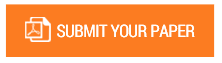Modeling and Performance Analysis of Armature-Controlled DC Motor

Authors : Nyein Nyein Soe, Aye Aye Maw.

Volume/Issue : Volume 4 - 2019, Issue 5 - May

Scribd : https://bit.ly/2HMbtjV

In this paper, performance analysis of dc motor is described. The selected dc motor is armaturecontrolled motor with fixed field. Moment of inertia with damper (friction) is connected directly to the dc motor as mechanical load. From the dynamic equations of this system to state space form, the roots of the system are evaluated and determine the motor is stable or unstable in open-loop operation. Mathematical modeling and Transfer Function modeling of the dc motor, with and without load torque are built by using MATLAB Simulink Software. Armature current, speed and electromagnetic torque of dc motor are simulated as the output results. This paper is mainly focus to apply MATLAB Software to the operation and modeling of fixed field dc motor in open-loop operation.

Keywords : DC Motor; Open-Loop Operation; Mathematical Modeling; Transfer Function Modeling; MATLAB Simulink Software.

#### CALL FOR PAPERS

Paper Submission Last Date
31 - March - 2023